Guan, C., Zhang, H., Hunt, J. F., Zhou, L., and Feng, D. (2016). "Measurement of dynamic viscoelasticity of full-size wood composite panels using a vibration testing method," BioRes. 11(2), 4593-4604.

#### Abstract

The dynamic viscoelasticity of full-size wood composite panels (WCPs) under the free-free vibrational state were determined by a vibration testing method. Vibration detection tests were performed on 194 pieces of three types of full-size WCPs (particleboard, medium density fiberboard, and plywood (PW)). The dynamic viscoelasticity from smaller specimens cut from the panels was measured using a cantilever beam vibration test apparatus, and the two data sets were compared. A strong linear relationship was discovered between the dynamic viscoelasticity values measured by the vibration detection test and the cantilever beam vibration test. The storage modulus values of the panels were far higher than their loss modulus values, and PW panels had the smallest value of loss modulus. For the panels tested, density had a good linear impact on storage modulus. In comparison with density, logarithmic decrement had a greater linear impact on loss modulus. This study demonstrated that the vibration test method is a valid approach for determining the dynamic viscoelasticity of full-size WCPs.

Measurement of Dynamic Viscoelasticity of Full-Size Wood Composite Panels Using a Vibration Testing Method

Cheng Guan,a Houjiang Zhang,a,* John F. Hunt,b Lujing Zhou,and Dan Feng a

The dynamic viscoelasticity of full-size wood composite panels (WCPs) under the free-free vibrational state were determined by a vibration testing method. Vibration detection tests were performed on 194 pieces of three types of full-size WCPs (particleboard, medium density fiberboard, and plywood (PW)). The dynamic viscoelasticity from smaller specimens cut from the panels was measured using a cantilever beam vibration test apparatus, and the two data sets were compared. A strong linear relationship was discovered between the dynamic viscoelasticity values measured by the vibration detection test and the cantilever beam vibration test. The storage modulus values of the panels were far higher than their loss modulus values, and PW panels had the smallest value of loss modulus. For the panels tested, density had a good linear impact on storage modulus. In comparison with density, logarithmic decrement had a greater linear impact on loss modulus. This study demonstrated that the vibration test method is a valid approach for determining the dynamic viscoelasticity of full-size WCPs.

Key words: Full-size wood composite panel; Dynamic viscoelasticity; Storage modulus; Loss modulus; Vibration testing

Contact information: a: School of Technology, Beijing Forestry University, Beijing 100083, China; b: USDA Forest Products Laboratory, 1 Gifford Pinchot Drive, Madison, WI, 53726-2398, USA;

*Corresponding author: hjzhang6@bjfu.edu.cn

INTRODUCTION

Engineered wood composite panels (WCPs) are widely used in furniture production, construction, packaging, transportation, musical instruments, and other sectors. Full-size WCPs refer to panels with a nominal size (length × width) of 2440 mm × 1220 mm, which are the most common in production and sales. To make use of full-size WCPs reasonably and effectively, accurate measurements of their mechanical properties are essential; thus, these measurements have been the focus of research for many years.

Modulus of elasticity (MOE) and shear modulus are two key indexes for evaluating the mechanical performance of WCPs. Conventionally, non-destructive techniques have been used to determine these performance parameters in WCPs. Most studies conduct non-destructive testing of MOE and shear modulus of small wood composites specimens and full-size WCPs, with remarkable results (McNatt et al. 1990; Shyamasunder et al. 1993; Larsson 1996; Yoshihara 2011; Wang et al. 2012; Hunt et al. 2013; Guan et al. 2015). However, similar to wood material, WCPs also exhibit dynamic viscoelastic effects. Dynamic viscoelasticity mainly refers to hysteresis phenomena and mechanical losses under the action of cyclic stress and strain. Its main parameter indicators include storage modulus and loss modulus. Storage modulus reflects the material’s elastic stiffness, and loss modulus reflects the damping properties of the material. Both moduli are important reference indicators for determining the mechanical properties of the material itself that affects the use of WCPs. Reports on the dynamic viscoelasticity on full-size WCPs are relatively rare. Current test equipment, such as dynamic mechanical analyzers (DMA), are generally limited to smaller samples with a thickness of no more than 10 mm. For thinner materials, the dynamic viscoelasticity of two types of hardboard have been tested using the vibrating reed method by means of non-destructive dynamic vibrations (Moslemi 1967). A cantilever beam apparatus was developed for determining dynamic viscoelasticity of wood or composite materials, and it quickly and accurately determines the dynamic viscoelasticity of small specimens of wood or WCPs (Yan 2010; Zhou et al. 2014b). Currently, there are no test methods or equipment that have been useful for determining the dynamic viscoelasticity for full-size WCPs.

Based on “free-free” vibration theory and support conditions, a laboratory test apparatus was developed to determine the dynamic MOE for full-size WCPs (Guan et al. 2015). This work is a continuation of initial research to determine the dynamic viscoelasticity of full-size WCPs using this apparatus. The objective of this paper is to examine the feasibility and validity of the vibration testing method for assessing dynamic viscoelasticity for full-size WCPs. Vibration detection tests were performed on 194 pieces of full-size WCPs. The panels tested included particleboard (PB), medium density fiberboard (MDF), and plywood (PW), over a range of thicknesses and densities. To examine the validity of this method, the data was compared with dynamic viscoelasticity measurements obtained from cantilever beam vibration tests performed on specimens cut from the sample panels.

EXPERIMENTAL

Theoretical Basis

The dynamic viscoelasticity (E*) of the full-size WCP was determined using Eq. 1,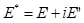(1)

where E is the storage modulus and E is the loss modulus.

“Free-free” support refers to the panel being supported on its two nodal lines, which are located at 22.4% and 77.6% of its length. A panel’s free vibration under this support state is called “free-free” support vibration (Guan et al. 2015). Both calculated modal analysis and experimental modal analysis show that the first vibration mode of a full-size panel under this support state is the first-order bending along the length direction of the panel, which is the same as the vibration mode of a beam supported at the same locations (Guan et al. 2014; Zhou et al. 2014a). Therefore, the related equation for storage modulus and loss modulus of the beam in this support state can be used to derive the equation for storage modulus, Eq. 2, and loss modulus, Eq. 3, of the panel (Guo and Liu 1985). The derived E’ and E” correspond to the storage modulus and loss modulus along the length direction of the panel, respectively,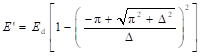(2)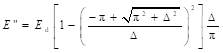(3)

where Ed is the dynamic MOE of the panel (Pa) and Δ is the logarithmic decrement of the panel.

According to the transverse vibration of a rectangular orthotropic plate, neglecting shear deformation and rotary inertia, the Ed of the panel can be calculated using Eq. 4 (Zhou and Chui 2015),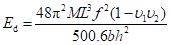(4)

where f is the natural first-order vibration frequency in bending along the length direction of the panel (Hz) without damping for this “free-free” support state, is the weight of the panel (kg), v1and v2 are the Poisson ratios of the panel, and Lbh are the length, width, and thickness of the panel (m), respectively.

The Poisson’s ratios of the panel have a very small influence on the first resonant frequency (Sobue and Kitazumi 1991; Schulte et al. 1996). Hence, substituting a constant value for the Poisson ratios will have a small influence on the calculated properties. Here v1v2 was assumed to be 0.01 as used for most wood materials (Hearmon 1946).

Free vibration of the panel in this support state appears as a damped sine wave. According to the damped sine wave vibration amplitude, the logarithmic decrement Δ is shown using Eq. 5 (Hunt et al. 2013),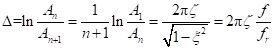(5)

where A1 is the first amplitude of the damped sine wave selected, Ais the nth amplitude of the damped sine wave selected, An+1 is the (n+1)th amplitude of the damped sine wave selected, f is first natural frequency of the panel vibration without damping, fr is first natural frequency of the panel vibration tested, and ζ is the damping ratio.

In Eq. 5, ζ can be calculated using the logarithmic decrement of vibrational decay (Δ) in Eq. 6: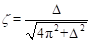(6)

According to Eq. 5 and Eq. 6, first natural frequency of the panel vibration (f) can then be calculated using the measured first frequency (fr), as shown in Eq. 7.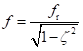(7)

When the panel geometry size (L, b, h) is given, the dynamic viscoelasticity values (storage modulus E and loss modulus E) for the full-size WCP are obtained by substituting these parameters into Eq. 2 and Eq. 3. This was the theoretical basis for determining dynamic viscoelasticity for the full-size WCP.

Materials

A total of 194 pieces of full-size WCPs were used in this study. There were 67 pieces of PB panels with different nominal thicknesses of 9, 16, 18, and 25 mm; 69 pieces of MDF panels with nominal thicknesses of 9, 12, 15, and 18 mm; and 58 pieces of PW panels with nominal thicknesses of 9, 15, 18, and 25 mm. The MDF panels were provided by a local MDF manufacturer (Krono, Beijing, China). These panels were manufactured from mixed species of both softwood and hardwood using urea-formaldehyde resin. The PB and PW panels were purchased from the local supplier (Beijing, China). So the resin types or other manufacturing characteristics were not available for this test. The PW panels were made from poplar veneer. Table 1 shows the specifications of the full-size WCP samples. The length (L), width (b), and thickness (h) of the panels were measured prior to testing. Average density of the panels was calculated based on the weight and volume. The moisture contents of the panels were determined using the National Standards of the People’s Republic of China GB/T 17657-2013 (2013).

Table 1. Dimensions and Physical Characteristics for the Full-size Wood Composite Panels Tested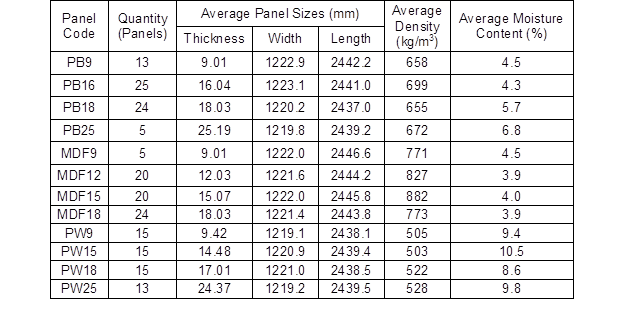After conducting the full size vibration test (described below), the measured panels were cut into smaller specimens for the cantilever beam vibration test. One specimen was cut from each full-size WCP along the length of the panel. As for the dimensions of the specimen, to ensure the effects of shear force and rotary motion in the specimen could be neglected, the ratio of free length to thickness were greater than 14.5 (Hunt et al. 2013). Detailed dimensions are shown in Table 2. The moisture content has a strong influence on material properties. Unfortunately, a laboratory with constant climate could not be used for the tests. To limit the influence of moisture content on comparison test results, there was a minimal time difference between the full-size tests and cantilever beam tests. The interval between the two tests was a maximum of 2 h for cutting specimens for the cantilever beam vibration test from the panel immediately after testing the full-size panel. The two tests, the full-size panel and the smaller cantilever beam test, were conducted in a room with a relative humidity of 30±5% and a temperature of 20±2 C.

Table 2. Dimensions for the Smaller Cantilever Beam Specimens Cut from the Full-size Panels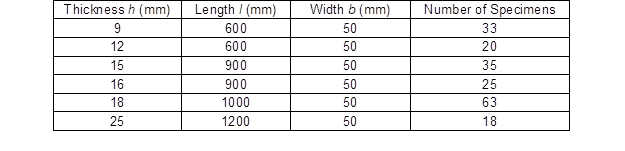Vibration Detection Test

The vibration detection test was conducted using the same laboratory testing apparatus for a previous study (Guan et al. 2015). Two load sensors were used to measure the weight of the full-size WCP being tested, and a laser sensor located at the middle of the panel was used to measure the vibrational displacement. LabVIEW software (Beijing, China) was written and used to collect and process both load and vibration signals and to calculate storage modulus and loss modulus based on Eq. 2 and Eq. 3 (Xu et al. 2009).

The full size panel was placed on the laboratory testing apparatus and was supported on bars at the locations, 22.4% and the 77.6% along its length direction. The testing software collected the load sensor signal and calculated the panel weight. An initial displacement was applied with both hands at one end of the panel away from the load sensor, and the hands were released from the end of the panel allowing the panel to vibrate at its free vibration state (first mode). The laser sensor recorded the vibration displacement signal used to calculate the first natural frequency (f) of the panel vibration and logarithmic decrement (Δ) of the vibrational decay. The software calculated the storage modulus (E) and loss modulus (E) of the panel. Through R language modeling, these test data were analyzed with regression analysis using one variant linear regression analysis method, analysis of variance, and t-test (Wang 2014).

Cantilever Beam Vibration Test

To examine the validity of the panel vibration testing method, the cantilever beam vibration test was conducted (Yan 2010; Zhou et al. 2014b). One end of each small specimen was clamped at a length of 50 mm. The free end of the specimen was displaced an initial distance and then released, resulting in re-vibration in the first mode of the cantilever beam. The cantilever vibration displacement signal was recorded. Storage modulus and loss modulus were calculated for each cantilever specimen.

RESULTS AND DISCUSSION

Dynamic Viscoelasticity for the Full-size WCPs

The average values and standard deviations (SD) for the storage modulus and loss modulus for all the panels are given in Table 3. The coefficient of variation (COV) and ratio of E to E of the three types of panels are also given in Table 3. The values for E were greater than the values for E for all panels tested, which was similar to previous studies of small specimens of hardboard, particleboard, and fiberboard (Moslemi 1967; Yan 2010; Zhou et al. 2014b). The ratios of their values were in the range of 24.94 to 77.61; the ratios of E and E of PB panels were in the range of 24.94 to 48.77. The ratios of E and E of MDF panels were in the range of 33.29 to 47.22, and the ratios of E and E of PW panels were in the range of 44.43 to 77.61. The ratios of E and Efor the PW panels were higher than the PB and MDF panels. Furthermore, the COV values of Ewere greater than those of E among the panels tested, which revealed that the variability of loss modulus was higher than that of the storage modulus for these three types of panels.

Table 3. Storage Modulus and Loss Modulus of Full-size Wood Composite Panels Tested Using Vibration Test Method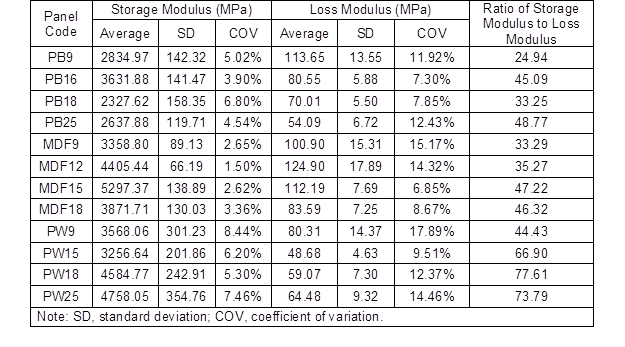Furthermore, PW panels had the lowest E values, indicating that PW panels exhibit lower dampening properties. This result is expected due to the cross-laminated veneer and higher stiffness properties or PW in general. Both PB and MDF panels have higher loss modulus values and are generally considered to have better damping properties for absorbing vibration energy. For example, PW panels are used to construct components in guitars and pianos that require improved resonance properties that have low loss modulus characteristics, whereas low-density MDF panels are used as room dividers to adsorb sound energy.

Density and Logarithmic Decrement Relationship with Dynamic Viscoelasticity

Relationship of storage modulus (E) with density

Figure 1 shows the relationship between E and density (ρ) for PB, MDF, and PW panels. The linear regression equations and the related parameters are listed in Table 4. There was a good linear relationship between E and ρ for the three types of panels, and their relationships were highly significant at the 0.001 level. Moreover, the correlation coefficients between E and ρ of PB panels, MDF panels, and PW panels were 0.883, 0.898, and 0.735, respectively.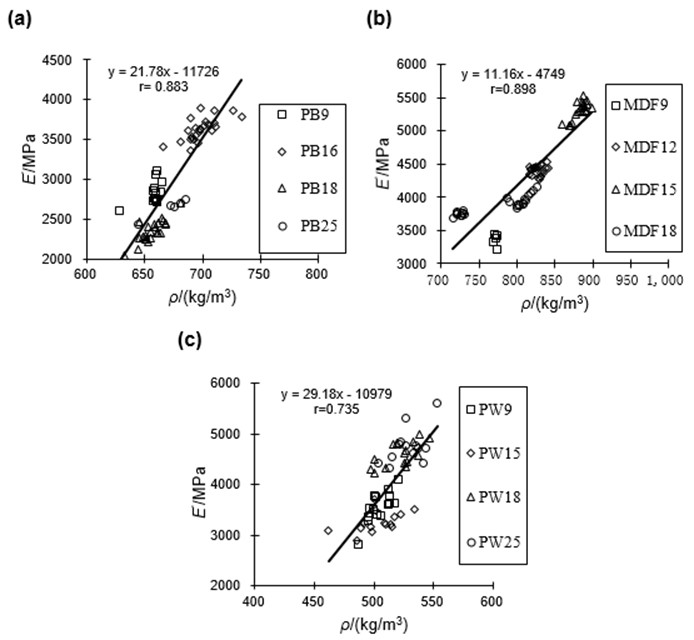Fig. 1. Relationship between storage modulus and density for full-size panels for (a) particleboard (PB), (b) medium density fiberboard (MDF), and (c) plywood (PW)

Table 4. Linear Regression Equations and Parameters Relating Storage Modulus with Density between Three Types of Panels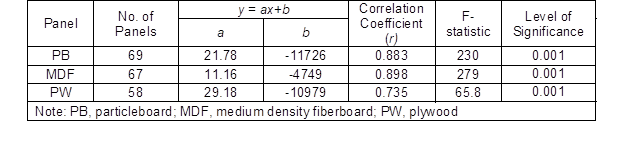Relationship of loss modulus (E) with logarithmic decrement

In the process of vibration, logarithmic decrement (Δ) is an important index for measuring material damping ratio. Figure 2 shows the relationship between E” and Δ among the three types of panels tested. Through the R language modeling, the test data was also analyzed in the same way as the E’ values. The linear regression equations and the related parameters are listed in Table 5. Figure 2 and Table 5 show that there was a positive linear relationship between E and Δ for these panels, which was highly significant at the 0.001 level. The correlation coefficients between E and Δ of PB, MDF, and PW panels were 0.720, 0.718, and 0.800, respectively.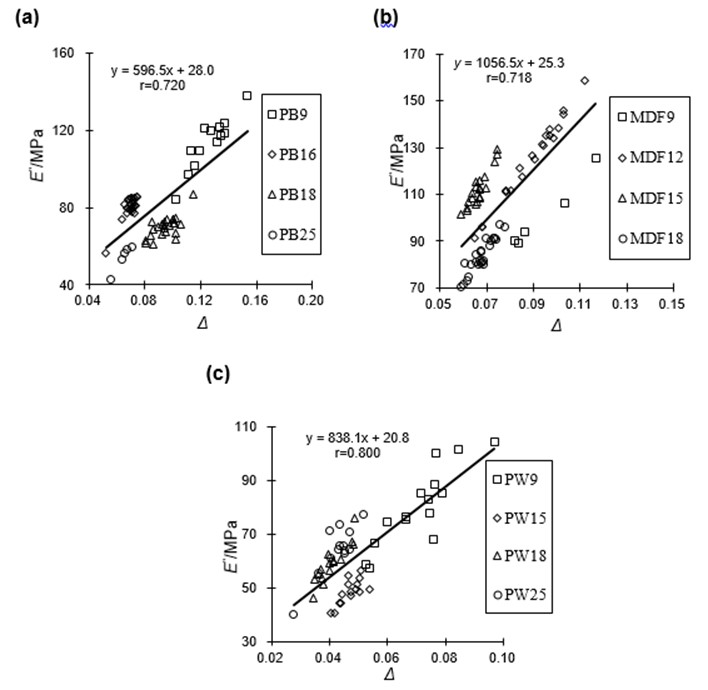Fig. 2. Relationship between loss modulus and logarithmic decrement for the three full-size WCPs (a) particleboard (PB), (b) medium density fiberboard (MDF), and (c) plywood (PW)

Table 5. Linear Regression Equations and Parameters Relating Loss Modulus with Logarithmic Decrement for the Three Types of Panels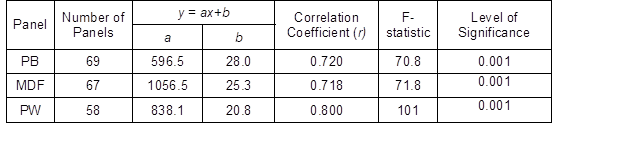Relationship of logarithmic decrement and density with loss modulus

In one variant linear regression analysis above, although there was a highly significant linear relationship between E and ρ as well as between E and Δ for the three types of panels tested, the correlation coefficient between E and Δ was not particularly high. Thus, a modified regression model was generated where Δ and ρ of the panels were selected as the independent variables x1and x2, and E was selected as the dependent variable y. Their linear regression equations and related parameters are listed in Table 6.

Table 6. Linear Regression Equations and Parameters Relating Loss Modulus with Logarithmic Decrement and Density between Three Types of Panels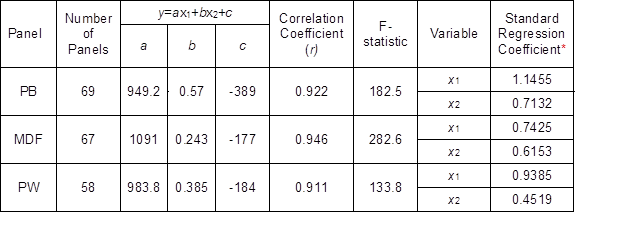*Level of significance = 0.001

The correlation coefficients between EΔ, and ρ among the types of panels tested were significantly higher than those between E and ρ in the one variant linear regression analysis discussed previously, which illustrated that a multiple regression model is meaningful for an automatic testing device. Moreover, the linear relationship between ρ and E as well as between Δand E were both highly significant at the 0.001 level; as observed from the standard regression coefficients, Δ had a greater effect than ρ on E.

Results Analysis of Dynamic Viscoelasticity in the Two Kinds of Methods

Comparisons with the cantilever beam vibration test were from only one small specimen cut from each panel. Due to possible poor uniformity of the panels tested, there were differences between the density of the panel and density of the small cantilever specimen. In terms of the analysis above, a good linear relationship was still found between E and ρ. However, to minimize the impact of a density difference between the small specimen and full-size WCP on the Evalues of small specimens, the impact of density on Es was accounted for by Eq. 8 (Moslemi 1967),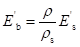(8)

where Es is the unadjusted storage modulus for the small specimen, Eb is the adjusted storage modulus of small specimen, and ρ and ρs are the average densities of full-size WCP and small specimen, respectively. The density ratio between full-size WCPs and corresponding small specimens ranged from 0.91 to 1.09.

A density difference between small specimen and full-size WCP can also lead to a damping ratio difference between the samples (Wang et al. 2012; Hunt et al. 2013). To eliminate the impact of a damping ratio difference between small specimen and full-size WCP on the Es of a small specimen, the impact of density and logarithmic decrement on Es was accounted for by Eq. 9,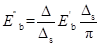(9)

where Es is the unadjusted loss modulus of small specimen, Eb is the adjusted loss modulus of small specimen, and Δ and Δare the logarithmic decrements of full-size WCP and small specimen, respectively.

After adjusting the dynamic viscoelasticity for the small specimen, the dynamic viscoelasticity of full-size WCPs and small specimens were compared (Fig. 3). There was a statistically significant, highly correlated linear relationship between storage modulus of all the panels tested and their small specimen. Similarly, the loss modulus was highly correlated with the small cantilever specimens. The linear correlations of storage modulus and loss modulus were 1.033 and 1.012, with the coefficients of correlation of 0.951 and 0.968, respectively, revealing that determining dynamic viscoelasticity of full-size WCPs by the vibration testing method was very effective and feasible.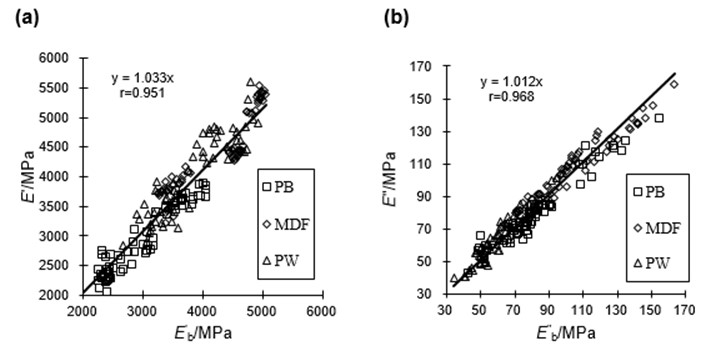Fig. 3. Relationship of dynamic viscoelastic properties between full-size panels (y-axis) and smaller cantilever beam specimen (x-axis) (a) storage modulus, and (b) loss modulus

CONCLUSIONS

1. The laboratory testing apparatus provides an easy method to determine dynamic viscoelasticity of full-size WCPs fast and accurately.
2. A statistically significant and highly correlated linear relationship existed between the dynamic viscoelasticity of the panels tested in the vibration detection test and small specimens tested using the cantilever beam vibration test.
3. Storage modulus values of PB, MDF, and PW panels were far higher than their loss modulus values, and PW panels had the smallest value of loss modulus.
4. For the panels tested, density had a good linear impact on storage modulus and logarithmic decrement had a greater linear effect on loss modulus compared with density.

ACKNOWLEDGMENTS

This project was funded by the State Forest Administration of China through the Special Research Funds for Public Welfare (Grant No. 201304512).

REFERENCES CITED

GB/T 17657-2013. (2013).Test Methods of Evaluating the Properties of Wood-based Panels and Surfaces Decorated Wood-based Panels, Standardization Administration of the People’s Republic of China (SAC), Beijing, China.

Guan, C., Zhou, L. J., Zhang, H. J., and Li, K. H. (2014). “Vibration modal analysis of the full-sized medium density fiberboard,” Applied Mechanics and Materials 620, 268-273.

Guan, C., Zhang, H. J., Zhou, L. J., and Wang, X. P. (2015). “Dynamic determination of modulus of elasticity of full-size wood composite panels using a vibration method,” Construction and Building Materials 100, 201-206. DOI: 10.1016/j.conbuildmat.2015.10.008

Guo, M. L., and Liu, S. X. (1985). Dynamic Performance Testing of Polymers and Composites, Shanghai Science and Technical Literature Press, Shanghai, China.

Hearmon, R. F. S. (1946). “The fundamental frequency of vibration of rectangular wood and plywood plates,” Proceedings of the Physical Society 58(1), 78-92.

Hunt, J. F., Zhang, H. J., Guo, Z. R., and Fu, F. (2013). “Cantilever beam static and dynamic response comparison with mid-point bending for thin MDF composite panels,” BioResources8(1), 115-129. DOI: 10.15376/biores.8.1.115-129

Larsson, D. (1996). “Stiffness characterization of wood based panels by modal testing,” in: 10th International Symposium on Non-destructive Testing of Wood, Lausanne, Switzerland, pp. 237-246.

McNatt, J. D., Wellwood, R. W., and Bach, L. (1990). “Relationships between small-specimens and large panel bending tests on structural wood-based panels,” Forest Products Journal 40(9), 10-16.

Moslemi, A. A. (1967). “Dynamic viscoelasticity in hardboard,” Forest Products Journal 17(1), 25-33.

Schulte, M., Frühwald, A., and Bröker, F. W. (1996). “Non-destructive testing of panel products by vibration technique,” in: 10th International Symposium on Nondestructive Testing of Wood, Lausanne, Switzerland, pp. 259-268.

Shyamasunder, K., Aswathanarayana, B. S., and Naidu, M. V. (1993). “Nondestructive evaluation of modulus of elasticity and modulus of rigidity of plywood by sonic methods,” in: Ninth International Symposium on Nondestructive Testing of Wood, Madison, USA, pp. 113-116.

Sobue, N., and Kitazumi, M. (1991). “Identification of power spectrum peaks of vibrating completely-free wood plates and moduli of elasticity measurements,” Mokuzai Gakkaishi 37(1), 9-15.

Wang, B. H. (2014). Multivariate Statistical Analysis and Modeling for R Language, Jinan University Press, Guangzhou, China.

Wang, Z., Li, L., and Gong, M. (2012). “Measurement of dynamic modulus of elasticity and damping ratio of wood-based composites using the cantilever beam vibration technique,” Construction and Building Materials 28(1), 831-834. DOI: 10.1016/j.conbuildmat.2011.09.001

Xu, X. D., Zhen, D. Y., and Xiao, W. (2009). LabVIEW 8.5 Commonly Using Functions and Programming Examples, Publishing House of Electronics Industry, Beijing, China.

Yan, H. C. (2010). Determining Dynamic Viscoelasticity and Shear Modulus of Wood Composites, Master’s Thesis, Beijing Forestry University, Beijing, China.

Yoshihara, H. (2011). “Measurement of the Young’s modulus and shear modulus of in-plane quasi-isotropic medium-density fiberboard by flexural vibration,” BioResources 6(4), 4871-4885. DOI: 10.15376/biores.6.4.4871-4885

Zhou, J. H., and Chui, Y. H. (2015). “Influence of boundary conditions on measurement of elastic constants of engineered wood-based panels using modal testing,” in: 19th International Symposium on Nondestructive Testing of Wood, Rio de Janeiro, Brazil, pp. 321-332.

Zhou, L. J., Zhang, H. J., Guan, C., and Wang, X. P. (2014a). “Analysis of vibration modal testing for the full-size artificial board,” Journal of Multimedia 9(6), 816-821. DOI: 10.4304/jmm.9.6.816-821

Zhou, L. J., Zhang, H. J., Yan, H. C., and Liao, C. H. (2014b). “Detection on the dynamic viscoelasticity of thin wood composites based on cantilever-beam free vibration,” Journal of Nanjing Forestry University: Natural Sciences Edition 38(2), 26-30. DOI: 10.3969/j.issn.1000-2006.2014.02.006.

Article submitted: January 24, 2016; Peer review completed: March 18, 2016; Revised version received: March 26, 2016; Accepted: March 30, 2016; Published: April 6, 2016.

DOI: 10.15376/biores.11.2.4593-4604